# MP Board Class 7th Maths Solutions Chapter 11 Perimeter and Area Ex 11.4

## MP Board Class 7th Maths Solutions Chapter 11 Perimeter and Area Ex 11.4

Question 1.
A garden is 90 m long and 75 m broad. A path 5 m wide is to be built outside and around it. Find the area of the path. Also find the area of the garden in hectare.
Solution:
Length of garden (l) = 90 m
Breadth of garden (b) = 75 m
Area of garden = l × b = 90 × 75 = 6750 m2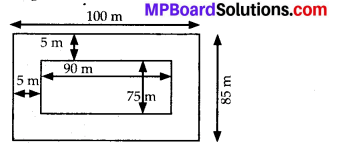From the figure, it can be observed that the new length and breadth of the garden, when path is also included, are (90 + 5 + 5) m i.e., 100 m and (75 + 5 + 5) m i.e., 85 m respectively.
Area of the garden including the path = 100 × 85 = 8500 m2
Area of path = Area of the garden including the path – Area of garden
= 8500 – 6750 = 1750 m2
1 hectare = 10000 m2
Therefore, area of garden in hectare = $$\frac{6750}{10000}$$
= 0.675 hectare

Question 2.
A 3 m wide path runs outside and around a rectangular park of length 125 m and breadth 65 m. Find the area of the path.
Solution:
Length of park (I) = 125 m
Breadth of park (b) = 65 m
Area of park = l × b = 125 × 65 = 8125 m2From the figure, it can be observed that the new length and breadth of the park, when path is also included, are (125 + 3 + 3) m i.e., 131 m and (65 + 3 + 3) m i.e., 71 m respectively.
Area of the park including the path = 131 × 71 = 9301 m2
Area of path = Area of the park including the path – Area of park
= 9301 – 8125 = 1176 m2

Question 3.
A picture is painted on a cardboard 8 cm long and 5 cm wide such that there is a margin of 1.5 cm along each of its sides. Find the total area of the margin.
Solution:
Length of cardboard (l) = 8 cm
Breadth of cardboard (b) = 5 cm
Area of cardboard = l × b = 8 × 5 = 40 cm2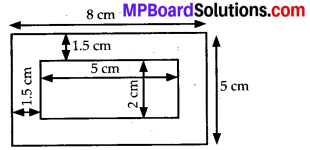From the figure, it can be observed that the new length and breadth of the cardboard, when margin is not included, are (8 – 1.5 – 1.5) cm i.e., 5 cm and (5 – 1.5 – 1.5) cm i.e., 2 cm respectively.
Area of the cardboard not including the margin = 5 × 2 = 10 cm2
Area of the margin = Area of cardboard – area of cardboard not including the margin
= 40 – 10 = 30 cm2Question 4.
A verandah of width 2.25 m is constructed all along outside a room which is 5.5 m long and 4 m wide. Find:
(i) the area of the verandah.
(ii) the cost of cementing the floor of the verandah at the rate of ₹ 200 per m2.
Solution:
(i) Length of room (l) = 5.5 m
Breadth of room (b) = 4 m
Area of room = l × b = 5.5 × 4 = 22 m2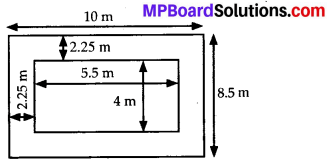From the figure, it can be observed that the new length and breadth of the room, when verandah is also included, are (5.5 + 2.25 + 2.25) m i.e., 10 m and (4 + 2.25 + 2.25) m i.e., 8.5 m respectively.
Area of the room including the verandah = 8.5 × 10 = 85 m2
Area of verandah = Area of the room including the verandah – Area of room = 85 – 22 = 63 m2

(ii) Cost of cementing 1 m2 area of the floor of the verandah = ₹ 200
Cost of cementing 63 m2 area of the floor of the verandah = 200 × 63 = ₹ 12600

Question 5.
A path 1 m wide is built along the border and inside a square garden of side 30 m. Find:
(i) the area of the path
(ii) the cost of planting grass in the remaining portion of the garden at the rate of ₹ 40 per m2.
Solution:
(i) Side of square garden (a) = 30 m
Area of square garden = a2 = (30)2 = 900 m2From the figure, it can be observed that the side of the square garden, when path is not included, is (30 – 1 – 1) m i.e., 28 m.
Area of the square garden not including the path = (28)2 = 784 m2
Area of path = Area of the square garden – Area of square garden not including the path = 900 – 784 = 116 m2

(ii) Cost of planting grass in 1 m2 area of the garden = ₹ 40
Cost of planting grass in 784 m2 area of the garden = 784 × 40 = ₹ 31360

Question 6.
Two cross roads, each of width 10 m, cut at right angles through the centre of a rectangular park of length 700 m and breadth 300 m and parallel to its sides. Find the area of the roads. Also find the area of the park excluding cross roads. Give the answer in hectares.
Solution: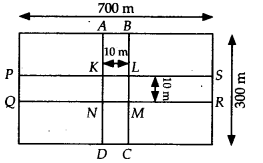Length of park (l) = 700 m
Breadth of park (b) = 300 m
Area of park = 700 × 300 = 210000 m2
Length of road PQRS = 700 m
Length of road ABCD = 300 m
Width of each road = 10 m.
KLMN is a square of side 10 m.
Area of two roads = area (PQRS) + area (ABCD) – area (KLMN)
= (700 × 10) + (300 × 10) – (10 × 10)
= 7000 + 3000 – 100 = 10000 – 100 = 9900 m2
= $$\frac{9900}{10000}$$ hectare = 0.99 hectare (∵ 1 hectare = 10000 m2)
Area of park excluding roads = 210000 – 9900
= 200100 m2 = $$\frac{200100}{10000}$$ hectare = 20.01 hectareQuestion 7.
Through a rectangular field of length 90 m and breadth 60 m, two roads are constructed which are parallel to the sides and cut each other at right angles through the centre of the fields. If the width of each road is 3 m, find
(i) the area covered by the roads.
(ii) the cost of constructing the roads at the rate of ₹ 110 per m2.
Solution: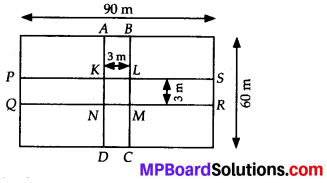Length of field (l) = 90 m
Breadth of field (b) = 60 m
Area of field = 90 × 60 = 5400 m2
Length of road PQRS = 90 m
Length of road ABCD = 60 m
Width of each road = 3 m
KLMN is a square of side 3 m.
(i) Area of the roads = area (PQRS) + area (ABCD) – area (KLMN)
= (90 × 3) + (60 × 3) – (3 × 3)
= 270 + 180 – 9 = 441 m2
(ii) Cost for constructing 1 m2 road = ₹ 110
Cost for constructing 441 m2 road
= 110 × 441 = ₹ 48510

Question 8.
Pragya wrapped a cord around a circular pipe of radius 4 cm (adjoining figure) and cut off the length required of the cord. Then she wrapped it around a square box of side 4 cm (also shown). Did she have any cord left? (π = 3.14)Solution:
Perimeter of circular pipe = 2πr
= 2 × 3.14 × 4 = 25.12 cm
Perimeter of the square = 4 × Side of the square = 4 × 4 = 16 cm
Length of cord left withPfagya = 25.12 – 16 = 9.12 cm

Question 9.
The adjoining figure represents a rectangular lawn with a circular flower bed in the middle. Find:(i) the area of the whole land
(ii) the area of the flower bed
(iii) the area of the lawn excluding the area of the flower bed
(iv) the circumference of the flower bed.
Solution:
(i) Area of whole land = Length × Breadth
= 10 × 5 = 50 m2
(ii) Area of flower bed = πr2 = 3.14 × 2 × 2
= 12.56 m2
(iii) Area of lawn excluding the flower bed = Area of whole land – Area of flower bed
= 50 – 12.56 = 37.44 m2
(iv) Circumference of the flower bed = 2πr
= 2 × 3.14 × 2 = 12.56 mQuestion 10.
In the following figures( find the area of the shaded portions: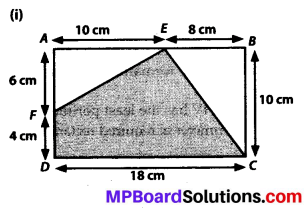Solution:
(i) Area of EFDC
= area(ABCD) – area (BCE) – area (AFE)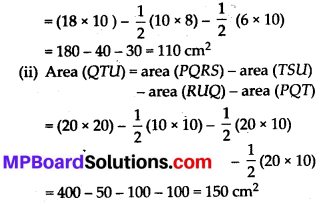Question 11.
Find the area of the quadrilateral ABCD.Here, AC = 22 cm, BM = 3 cm, DN = 3 cm and BM⊥AC, DN⊥AC
Solution:
Area (ABCD) = area (ABC) + area (ADC)
= $$\frac{1}{2}$$ (3 × 22) + $$\frac{1}{2}$$ (3 × 22)
= 33 + 33 = 66 cm2